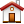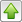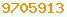# Floating Point : Java Glossary

Floating Point
By floating point I refer generically to the code written to use the primitives double and float and the wrapper classes Double and Float. Every person encountering floating point arithmetic is astonished. Java’s floating point is much better behaved that floating point implementations of yesteryear, but it still rarely fails to astonish. My general rule is, if at all possible, avoid floating point. Operations are usually faster with integers and certainly more predictable for the naïve programmer. Unless you have a deep understanding of what is going on inside IEEE (Institute of Electrical & Electronics Engineers) arithmetic, it is best to pretend a demon comes along at the end of your computation and adds a small signed error to any result. Here are some of the points for novices to grasp:
1. ## Inaccuracy

Think of float and double as representing physical measurements. No one would complain if their cabinet maker made a desk 6.000000000001 feet long. Analogously, don’t complain about the inevitable tiny errors in floating point arithmetic results e.g. Math. cos( Math.toRadians( 90 ) ) not coming out bang on zero. ( If you want perfection, use int, long, BigInteger or BigDecimal. )
2. ## Conversion

To interconvert int, long, float, double, Float and Double see the Conversion Amanuensis.
3. ## Consequences of Binary Arithmetic

The computer floating point unit works internally in base 2, binary. The decimal fraction 0.1 cannot be precisely represented in binary. It is a repeater fraction 0.00011001100110… It is like the repeater fraction 1/3 = 0.33333 in base 10. When you add 0.333333… to 0.666666… why are you not astonished to get 0.999999… rather than 1.0, even though you just added 1/3 + 2/3 to get 1? Yet, with Java floating point you are astonished when you add 0.1 + 0.1 and get something other than 0.2. The same fundamental mathematical cause is at work. It is God’s fault for not making decimal 0.1 a perfect fraction in binary notation. Mike Cowlishaw, the creator of NetRexx, blames computer hardware makers for using binary floating point. He figures computer chips should use decimal notation like humans. 1/3 is also a repeater in binary, 0.01010101010… so it too will misbehave in Java just the way it does with decimal arithmetic.
4. ## Slop

Floating point is by its nature inexact. It is probably best if you imagined that after every floating point operation, a little demon came in and added or subtracted a tiny number to fuzz the low order bits of your result. This is, of course, not true, but if you as newbie act as if it were true, this will lead you to code conservatively and will keep you out of trouble.

What actually happens is the computer only has 64 bits to work with. It has to throw away the low order part of any result after every operation. On every calculation you accumulate a little more roundoff error. In the newer Java’s the computer is permitted to secretly retain a few extra bits of accuracy during a short string of calculation, so sometimes they come out more accurate that you would theoretically expect. (They almost never come out more accurate than a newbie expects.) To discourage use of these guard bits and get reproducibly less accurate results you use the StrictMath library. Unless you really know what you are doing, you must presume the results are never precisely bang on. Don’t count on results that in theory should be integers coming out precisely as integers. Never compare == or !=, check within a tolerance. Keep in mind when you compare > >= < <= the values you are comparing may, as a side effect of calculation, have drifted just above or just below your test limits. Sometimes you may want to include some slop/tolerance in your limits. Java is somewhat better than other languages since it specifies strict IEEE rules. Fortunately, in Java, if a number has a perfect integer floating point representation and you divide it by another such number that is a factor, the result is guaranteed to be a perfect integer representation.

The other source of the fuzz is accumulating roundoff error from cascaded operations. Further, there are errors in trig functions not being bang on 0 or 1 as you expect. This is a result of accumulated roundoff error in evaluating polynomials and in the polynomial approximations themselves used internally to compute the trig functions.

For example, Math.E = 2.718281828459045 but Math. exp( 1.0 ) = 2.7182818284590455

5. ## Converting to String

When numbers are converted from float or double to String for display they may be truncated. Further, the process of converting from binary to decimal introduces even more errors that were not in the original computation result, possibly because of repeaters — fractions that cannot be represented exactly in binary or decimal. For finer control of number of decimal places, lead zeros, sign etc. see DecimalFormat.
6. ## Maintaining Precision

How can you bypass this fundamental inaccuracy?
• ### Approximate Comparison

Do everything with float/double, but whenever you do a compare, realise there will be some slop in the answer. So instead of asking
`if ( f == 100.00 )`
say
`if ( f > 99.995 )`
• ### Equality Testing

You can do testing for floating point equality like this:
`if ( Math.abs( value - target ) < epsilon )`
or
`if ( value >= target - epsilon && value <= target + epsilon )`

when the order of magnitude of target is unknown, you might use some slower code like this, presuming

`if ( Math.abs( value - target ) < epsilon * target )`
or
`if ( value >= target * ( 1 - epsilon ) && value <= target * ( 1+ epsilon ) )`
• ### Loops

Use a mixture of ints and float /doubles. Use the ints for your loop counting.

Instead of incrementing a floating point variable, recreate it from an int loop variable, e. g. instead of:

`f += 0.001;`
code
`f = i * 0.001;`
• ### double vs float

Use double instead of float. This helps but does not totally solve the problem. All integers less than 2^53 (roughly 16 digits) can be exactly representable in double. This does not mean that results of a calculation that theoretically should be an integer will actually be one, unless the IEEE spec demands it.
• ### Perfect Accuracy

When you want exact results, you must use ints, longs, BigDecimal (arbitrary precision decimal fractions) or BigIntegers (arbitrary precision Integers). Currency is best handled by storing pennies and adding a decorative decimal point on display.
currency for more details
7. ## How Precise?

32-bit floats can accurately represent ints up to 24 bits; 64-bit doubles represent longs up to 53 bits; 80-bit extended reals (when guard bits are permitted) represent longs up to 64 bits. This does not mean than necessarily every operation you where you expect an integer result will always give one. Arithmetic is guaranteed to be rounded to the nearest possible floating point representable number. However, as I have said before, not every number in representable and in particular fractions 0.1 0.01 etc. are not. It is best to presume it could be a tiny bit off, unless you fully understand the IEEE rules and can predict when you can count on accurate results. Happily Math.sqrt will give precise integer results when they can be precisely represented.
8. ## Binary Formats

To study the IEEE format, you can use Double.doubleToLongBits and Double.longBitsToDouble.

9. ## Math Dot

The rest of the world says y=sin(x);. Java (and JavaScript) insists that you say y=Math. sin(x); unless you are using Java version 1.5 or later and you say:
import static java.lang. Math.sin ; at the top.
10. ## String to float

The unusual thing about Java in this area is the high precision of its default String conversion for floating point values. Most languages by default round to some reasonable number of digits. When you convert an internal binary double number for display with:
`String Double.toString( double )`
Java wants to preserve every drop of precision it has, so that if you convert it back with:
`double Double.parseDouble( String )`
you will get back to the precise same binary representation. This is all very well, but from the point of view of humans, the display looks wrong, as if result were slightly inexact and with way too many digits. This also makes the rounding error distressingly visible. To fix it and create something that looks pleasingly rounded to humans, use java.text.DecimalFormat to limit the number of digits displayed to what you actually want/need.

If you are merely trying to round for internal purposes use Math.round, Math.floor, Math.ceil and Math.rint. Math.round gives a long result, the rest double. You can create variants by adding .5 and multiplying and dividing by powers of ten. There is generally no need to convert to int or long and back.

11. ## Platform differences

Not all implementations conform to the accuracy specs.
12. ## Speed

In most computers, floating point arithmetic is usually much slower than integer arithmetic, though on the Intel Pentium it is usually faster because the integer unit was not given the same care as the floating point unit.
Comparison of Pentium Floating Point and Integer Speeds
Operation Floating Point clocks Integer Clocks
multiply 1-3 10-11
division 39-42 22-46
convert 6 (double to long) 3 (long to double)
Floating point is slower still when you consider the overhead of converting between the combined int/floating point stack in the JVM (Java Virtual Machine) and the separate stacks in the Pentium hardware.

Double precision arithmetic has very little speed penalty on modern CPUs (Central Processing Units). Normally you should use double in preference to float. It gives you 14 to 15 significant digits where float gives only 6 to 7. The only advantage to float is compactness. In comparison, a typical scientific pocket calculator will give you 10 significant digits and will automatically round for display. When do you use float and when double? It depends how much precision you need. Because modern floating point hardware is all built on double, normally the only time you use float is for float[] or for float fields in plentiful Objects to conserve space when you don’t need the precision.

13. ## Nan

If you divide 0/0, 7/0 or overflow the maximum representable number, you won’t get an exception. You will get a special marker result. You can test for it with  Math.isInfinite or isNaN. NaN (Not A Number)s are strange beasts; they were included in the IEEE 754 standard so that the arithmetic would be algebraically complete, that is, so every operation on any set of arguments would have some IEEE 754 value as a result. NaNs therefore don’t follow the same rules as numeric values, even infinities. Odd NaN != NaN is true!! This gives you an easy and definitive way to test for a NaN. Ther would be no benefict to having Double.POSITIVE_INFINITY != Double.POSITIVE_INFINITY.

Other odd results you can get from floating point operations include Double. NEGATIVE_INFINITY, Double. POSITIVE_INFINITY and positive and negative 0. There is a similar method for checking for infinity, Double. isInfinite.

0./0. gives NaN, 1./0. gives POSITIVE_INFINITY and -1./0. gives NEGATIVE_INFINITY.

Because there are two different flavours of NAN, Double.POSITIVE_INFINITY and Double.NEGATIVE_INFINITY You can’t directly compare == Double.NAN to check for NAN. However, you can Double.isNaN or directly compare == Double.NAN; However, you can use == to compare with Double.POSITIVE_INFINITY. There is a corresponding Float. NaN and  Float.isNaN The theory is making NaN not equal to itself allows a quick and dirty way to test for a calculation going haywire.

```if ( result != result )
{
out.println( "oops" );
}```
14. ## Negative 0

IEEE format has two different bit patterns, one for positive 0 and one for negative 0. However, in Java, 0.d == -0.d. +/-0 is also used to represent any number too small to be directly represented in the IEEE range.
15. ## Powers

Math.pow works by taking a natural logarithm, multiplying and then taking the exp (anti-log). The log and exp are each computed by the CPU (Central Processing Unit) floating point microcode with a fat polynomial approximation (a great mess of multiplies and adds). This means if you want to compute a cube, you are best to use:
```// fast accurate way to cube a number
double y = x * x * x;```
rather
```// slow, inaccurate way to cube a number
double y = Math.pow( x, 3 );```
Ditto for squaring. Further, if x is an integer, you will get a precise result using only simple arithmetic. If you use: Math. pow you will lose precision. Also use
```// fast way to do a square root
double y = Math.sqrt( x );```
in
```// slow way to do a square root
double y = Math.pow( x, 0.5 );```

## Calculating

Here is how to do common non-trigonometric floating point calculations:
Common Floating Point Calculation
Method Purpose
- subtraction.
* mulitplication.
/ division, with a fractional result. If you divide by 0/0, 7/0 or overflow the maximum representable number, you won’t get an exception. You will get a special marker result. You can test for it with Math. isInfinite or isNaN.
Oracle’s Javadoc on Math.abs : available:
absolute value.
Oracle’s Javadoc on Math.cbrt : available:
cube root.
Oracle’s Javadoc on Math.ceil : available:
ceiling, next highest integer.
Oracle’s Javadoc on Math.exp : available:
ex.
```// compute exponent if number were written in scientific notatation
int exponent = (int) (Math.floor( Math.log10( value ))) + 1;
// e.g. 12345.0 ->  0.12345E06 -> exponent 6, mantissa  0.123454.
// Regular (int) rounds toward 0.```
base 10 exponent were the number written in scrientific notation. Internally number use IEEE binary exponents. Can also be used to determine the number of places to the left or right of the decimal point.
Oracle’s Javadoc on Math.hypot : available:
hypotenuse, distance from origin to a point: sqrt( x² + y² ). Note if you want to do is compare two distances, you don’t need the sqrt.
Oracle’s Javadoc on Math.log : available:
natural log, base e log, ln. Not base 10 common logarithm! Math.log gives: 0.01-4.6051, 0.1-2.3026, 1.00.0, 10.02.3026, 100.04.6052.
Oracle’s Javadoc on Math.log10 : available:
base 10 log. Math.log10 gives: 0.01-2.0, 0.1-1.0, 1.00.0, 10.01.0, 32.01.50514, 50.01.69897, 100.02.0.
```// compute mantissa if number were written in scientific notatation
int exponent = (int)(math.floor( Math.log10( value ))) + 1;
double mantissa = value * Math.pow( 10.0, -exponent );
// e.g. 12345.0 ->  0.12345E06 -> exponent 6, mantissa  0.123454.
// regular (int) rounds toward 0.```
base 10 mantissa were the number written in scientific notation. Internally Java uses IEEE binary exponents.
Oracle’s Javadoc on Math.max : available:
larger of two numbers.
Oracle’s Javadoc on Math.min : available:
smaller of two numbers.
Oracle’s Javadoc on Math.pow : available:
power, ab.
Oracle’s Javadoc on Math.rint : available:
round to nearest integer. Result is a double that is an integer.
Oracle’s Javadoc on Math.round : available:
round to nearest integer. Result is an long.
Oracle’s Javadoc on Math.signum : available:
Returns the signum function of the argument; 0 if == 0, 1.0 if > 0, -1.0 if < 0. The result is a double, not an int as you would expect.
Oracle’s Javadoc on Math.sqrt : available:
square root.

## Learning More

Oracle’s Javadoc on java.lang.Math class : available:
Oracle’s Javadoc on Double.isNaN : available:
Oracle’s Javadoc on Double.isInfinite : available:
Oracle’s Javadoc on Double.doubleToLongBits : available:
Oracle’s Javadoc on Double.longBitsToDouble : available:This page is postedon the web at: http://mindprod.com/jgloss/floatingpoint.html Optional Replicator mirror of mindprod.com on local hard disk J: J:\mindprod\jgloss\floatingpoint.htmlPlease read the feedback from other visitors, or send your own feedback about the site.Contact Roedy. Please feel free to link to this page without explicit permission. Canadian Mind Products IP:[65.110.21.43] Your face IP:[3.80.4.76] Feedback You are visitor number